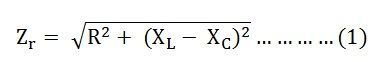# Series Resonance

In the RLC series circuit, when the circuit current is in phase with the applied voltage, the circuit is said to be in Series Resonance. The resonance condition arises in the series RLC circuit when the inductive reactance is equal to the capacitive reactance.

XL = XC or (XL – XC = 0)

A series resonant circuit has the capability to draw heavy current and power from the mains; it is also called acceptor circuit. The series resonance RLC circuit is shown in the figure below:At the resonance : XL – XC = 0 or XL = XC

The Impedance will be:Where Zr is the resonance impedance of the circuit.

Putting the value of XL – XC = 0 in equation (1) we will get:

Zr = R

Current I = V/ Zr = V/R

Since at resonance the opposition to the flow of current is only resistance (R) of the circuit. At this condition, the circuit draws the maximum current.

Also See: What is Resonant Frequency?

## Effects of Series Resonance

The following effects of the series resonance condition are given below:

• At resonance condition, XL = XC the impedance of the circuit is minimum and is reduced to the resistance of the circuit. i.e Zr = R
• At the resonance condition, as the impedance of the circuit is minimum, the current in the circuit is maximum. i.e Ir = V/Zr = V/R
• As the value of resonant current Ir is maximum hence, the power drawn by the circuit is also maximized. i.e Pr = I2Rr
• At the resonant condition, the current drawn by the circuit is very large or we can say that the maximum current is drawn. Therefore, the voltage drop across the inductance L i.e (VL = IXL = I x 2πfrL) and the capacitance C i.e (VC = IXC = I x I/2πfrC) will also be very large.

In the power system, at the resonant condition, the excessive voltage is built up across the inductive and capacitive component of the circuit such as circuit breaker, reactors, etc., may cause damage. Therefore, the series resonant condition is avoided in the power system.

However, in some of the electronics devices such as antenna circuit of radio and TV receiver, tunning circuit, etc. The series resonant condition is used to increase the signal voltage and current at the desired frequency (fr).

### 1 thought on “Series Resonance”

1. look guys I just want to say Thank You, I really mean it. This topic was really a pain but now its like I got the correct medicine. Thank you for the healing portion guys.